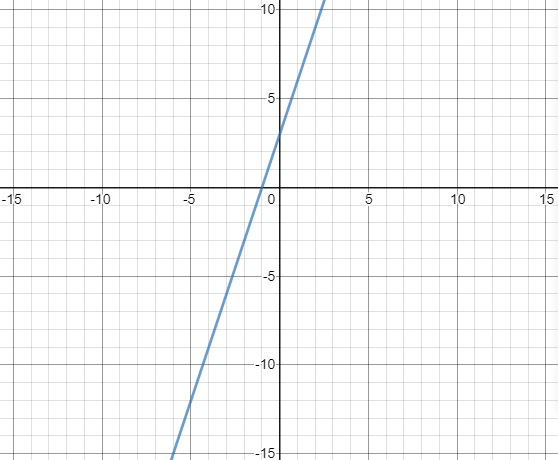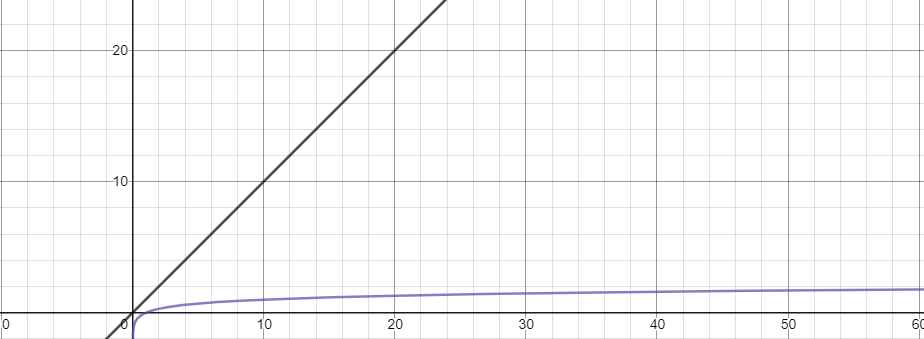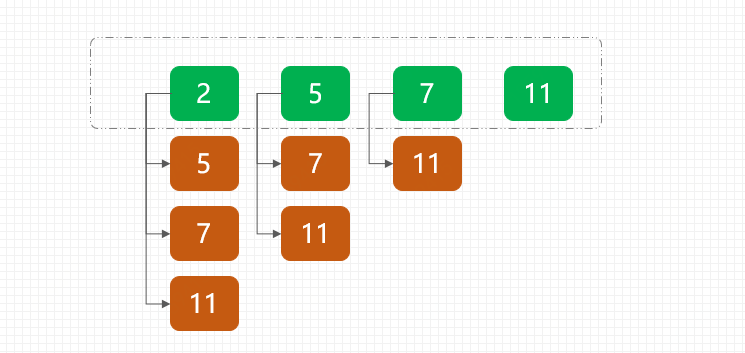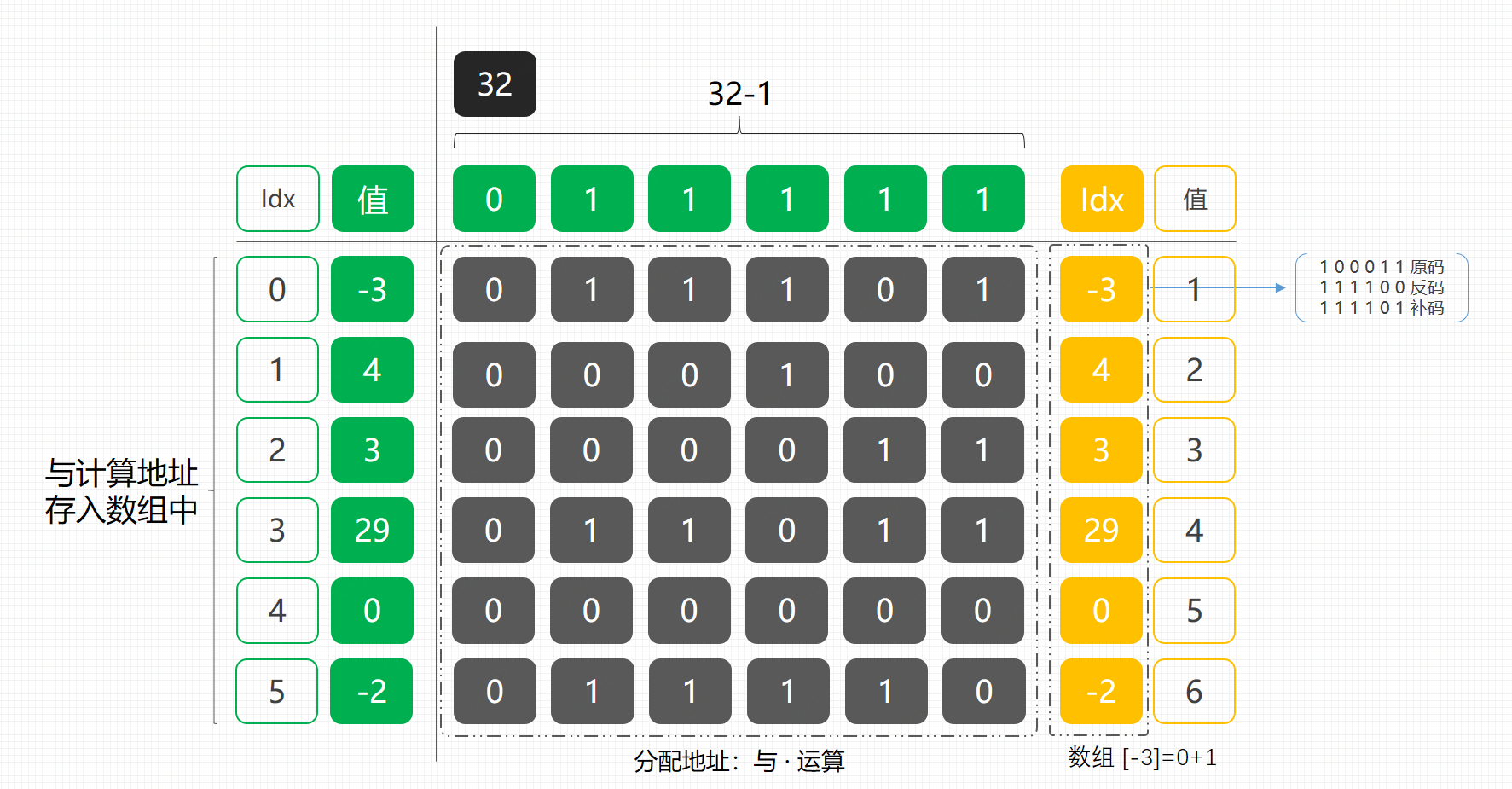# 野路子搞算法《两数之和》，带着小白刷面试算法题

2020/03/14

Github： https://github.com/MyGitBooks/niubility-algorithm

## 一、前言

leetcode-cn.com 中每个算法题都有编号；`1 2 3 ... 1566`，而且还在增加。你我都是新人，既然没了解过那就从第一题开始吧，尝试从算法中吸取一些创新的思路。否则为什么那么多公司面试招聘都会去考下算法！`谷歌` `字节跳动` `腾讯` `阿里` `等等`

## 二、时间复杂度

### 1. O(n)

``````int n = 10;
for (int i = 0; i < n; i++) {
System.out.println(i);
}
``````

1 int n = 10 1
2 int i = 0 1
3 i < n n + 1
4 i++ n
5 System.out.println(i) n

``````sum = 1 + 1 + n + 1+ n + n
= 3n+3
= n (忽略低阶梯)
``````### 2. O(logn)

``````int sum = 1, n = 10;
while (sum < n) {
sum = sum * 2;
}
``````## 三、算法题：两数之和

https://leetcode-cn.com/problems/two-sum/submissions/

``````给定 nums = [2, 7, 11, 15], target = 9

``````

java

``````class Solution {
public int[] twoSum(int[] nums, int target) {
// todo
}
}
``````

## 四、解题

### 思路1，双层循环``````public int[] twoSum(int[] nums, int target) {
int[] idxs = new int;
for (int i = 0; i < nums.length; i++) {
for (int j = i + 1; j < nums.length; j++) {
if (nums[i] + nums[j] == target) {
idxs = i;
idxs = j;
}
}
}
return idxs;
}
``````• 对于这样的算法虽然能解决问题，但是并不能满足我们的需求，毕竟这个级别的时间复杂度下实在是太慢了。

### 思路2，单层循环• 这个过程的核心内容是将原来的两数之和改成差值计算，并将每次的差与 Map 中元素进行比对。如果差值正好存在 Map 中，那么直接取出。否则将数存入到 Map 中，继续执行。
• 这个过程就可以将原来的双层循环改为单层，时间复杂度也到了 O(n) 级别。

``````public static int[] twoSum(int[] nums, int target) {
Map<Integer, Integer> hashMap = new HashMap<Integer, Integer>(nums.length);
for (int i = 0; i < nums.length; i++) {
if (hashMap.containsKey(target - nums[i])) {
return new int[]{hashMap.get(target - nums[i]), i};
}
hashMap.put(nums[i], i);
}
return new int[]{-1, -1};
}
``````• 可以看到当我们使用 Map 结构的时候，整个执行执行用时已经有了很大的改善。但是你有考虑过`containsKey``get` 是否为 null 相比哪个快吗？
• 这个算法已经很良好了，但是这个对 key 值的比对还是很耗时的，需要反复的对 map 进行操作，那么我们还需要再优化一下。

### 思路3，Bit结构• 左侧是我们假定的入参`int[] nums`，32是我们设定的值，这个值的设定需要满足存放大小够用，否则地址会混乱。
• 接下来我们使用 32 - 1，也就是二进制 `011111`与每一个数组中的值进行与运算，求存放地址。
• 当算好地址后，将元素存放在数组中，设置值。这个值就是我们的元素本身位置了，但是需要`+1`，因为默认数组是0，如果不加1，就看不到位置了。最终使用的时候，可以再将位置结果 `-1`

``````public static int[] towSum(int[] nums, int target) {
int volume = 2048;
int bitMode = volume - 1;
int[] t = new int[volume];
for (int i = 0; i < nums.length; i++) {
int c = (target - nums[i]) & bitMode;
if (t[c] != 0) return new int[]{t[c] - 1, i};
t[nums[i] & bitMode] = i + 1;
}
return new int[]{-1, -1};
}
``````
• 这个2048是我们试出来的，主要根据leetcode中的单测用例决定。• 出现0毫秒耗时，100%击败，这个不一定每次都这样，可能你试的时候不一样。得益于数据结构的优化使得这个算法的耗时很少。

## 五、总结

• 野路子搞算法，没有看过算法导论、也没有套用模板，但如果需要后续的不断的加深自己的知识点，也是需要学习的。目前在我看来这些更像是数学题，主要可以提升对同一件事情的多种处理方式，同时也增加个人的编程能力。
• 算法的学习也不太应该套用各种理论，当然每个人看法不一样，我允许你的观点，也要接受我的想法。
• 在各个大厂面试过程中，都有；算法、源码、项目、技术栈以及个人的一些优点，如果你能在前两个点上给面试官很好的印象，那么你就放心的要工资吧。
• 从这篇文章开始，我会陆续做一做算法题，提升自己的功夫底子，也分析给小白。欢迎小白跟随！Git地址：https://github.com/MyGitBooks/niubility-algorithm

（转载本站文章请注明作者和出处 微信公众号：bugstack虫洞栈 | 作者：小傅哥